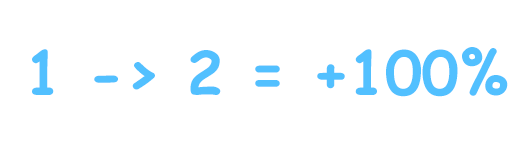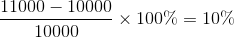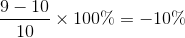# Percentage Calculator## Please enter the below information to calculate the percentage

Calculate what is how much % of a value
Calculate the percentage of a value over another value
Calculate the percentage increase or decrease
Information

## What is percentage?

Percentage is a way to express extent of a change for a variable. It represents the relative change between the old value and the new one.

For example:

My salary is \$10,000 dollars per month, my boss increase my salary to \$11,000 dollars, the percentage calculation is:The salary increased by 10%

Internet services fee changed from \$10 to \$9 per month, the percentage calculation is:Internet services fee decreased by 10%

This percentage calculator allows you to calculate percentage easily in mobile, without the use of MS Excel. If you like this small tool, please share! Thanks!